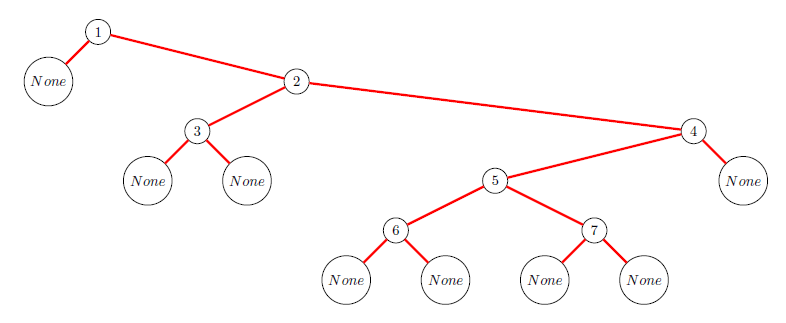# Revision history [back]

This is not an answer but a workaround in the case that one wishes a standalone picture.

Instead of ascii_art(t) execute pretty_print(t). Then edit the TeX code like this:

• Replace commands of the type

\newcommand{\nodec}{\node[draw,circle] (c) {\$None\$} by

\newcommand{\nodeb}{\node[draw,rectangle] (c) {$$} • Replace commands of the type \newcommand{\nodei}{\node[draw,circle] (i) {\6\} by \newcommand{\nodei}{\node[fill=black,draw,circle] (i) {$$}

• Replace

\matrix[column sep=.3cm, row sep=.3cm,ampersand replacement=\&] by

\matrix[row sep=0.7cm,ampersand replacement=\&]

• Replace

\path[ultra thick, red] by

\path[black]

This will replace the tree in Sage styleby a tree in Knuth style:This is not an answer but a workaround in the case that one wishes a standalone picture.

Instead of ascii_art(t) execute pretty_print(t). Then edit the TeX code like this:

• Replace commands of the type

\newcommand{\nodec}{\node[draw,circle] (c) {\$None\$} by

\newcommand{\nodeb}{\node[draw,rectangle] (c) {$$} • Replace commands of the type \newcommand{\nodei}{\node[draw,circle] (i) {\6\} by \newcommand{\nodei}{\node[fill=black,draw,circle] (i) {$$}

• Replace

\matrix[column sep=.3cm, row sep=.3cm,ampersand replacement=\&] by

\matrix[row sep=0.7cm,ampersand replacement=\&]

• Replace

\path[ultra thick, red] by

\path[black]

This will replace the tree in Sage styleby a tree in Knuth style:I really would like to see this as the default style for binary trees.